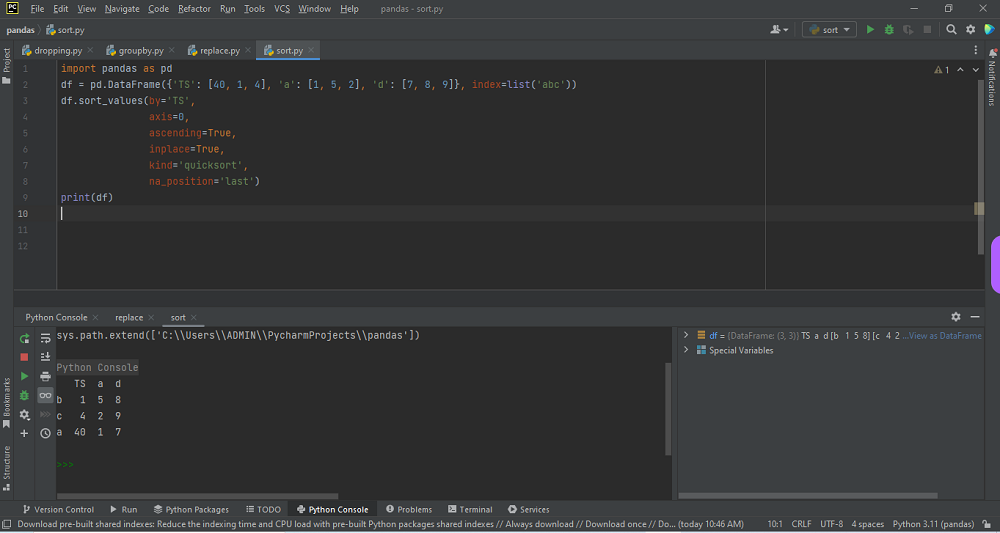# Pandas DataFrame sortingby vsasikalabeUpdated: Mar 6, 2023Solution Kit

By importing pandas as “pd,” we can create a data structure consisting of keys that identify the columns. Assign a set of values for each key that will populate each column. All the values are stored in a data frame. Within a data frame, sorting data can be executed using the sort_values() function.sorting in ascending order is the default value of this function. We can sort the data frame by using three categories:

• Sorting by index labels
• Sorting by column values
• Sorting by a combination of index labels and column values

In pandas, to sort the data frame sort_values() function is used. Using this method, we can sort the data frame in Ascending or Descending order. Firstly, let's create a Data Frame to represent a custom sort. This will return a new Data Frame sorted in ascending order by default. You must use ascending boolean property to specify the order. False for descending and True for ascending. By default, it is set to True.

The column will be sorted in descending order instead by passing in the ascending argument with a value of False. Use sort_values() and sort_index() to choose different sorting algorithms. We have three algorithms quicksort, mergesort, and heapsort. We can also take input tables or values from files using read_csv().

• By the values of one or more columns, we can Sort a pandas Data Frame.
• To change the sort order, use the ascending parameter.
• Using sort_index(), Sort a Data Frame by its index.
• We can find the missing data while sorting values.
• Using the inplace set to True, we can Sort a Data Frame in place.

Here is an example of how to perform Pandas Data frame Sorting:Preview of the output that you will get on running this code from your IDE.

### Code

In this solution we used pandas library of python.

### Instructions

Follow the steps carefully to get the output successfully:

1. Copy the code using the "Copy" button above, and paste it in a Python file in your IDE(Pycharm Preferable).
2. Run the file to generate the output.

I hope you found this useful. I have added the link to dependent libraries, version information in the following sections.

I found this code snippet by searching for "Pandas DataFrame sorting"in kandi. You can try any such use case!

### Environment Tested

I tested this solution in the following versions. Be mindful of changes when working with other versions.

1. The solution is created in Python 3.11.1 Version
2. The solution is tested on pandas 1.5.2 Version

Using this solution, we can do Pandas DataFrame sorting.This process also facilities an easy to use, hassle free method to create a hands-on working version of code in python which would help us to do Pandas DataFrame sorting.

### Dependent Library

pandasby pandas-dev

Python37415Version:v2.0.0rc1Flexible and powerful data analysis / manipulation library for Python, providing labeled data structures similar to R data.frame objects, statistical functions, and much more

Support
Quality
Security
Reuse

pandasby pandas-dev

Python37415Version:v2.0.0rc1License: Permissive (BSD-3-Clause)

Flexible and powerful data analysis / manipulation library for Python, providing labeled data structures similar to R data.frame objects, statistical functions, and much more
Support
Quality
Security